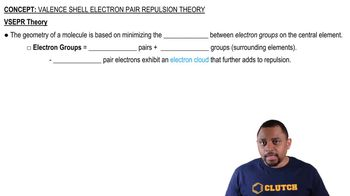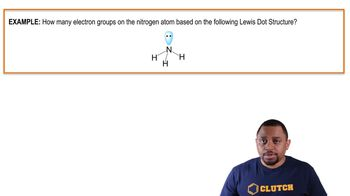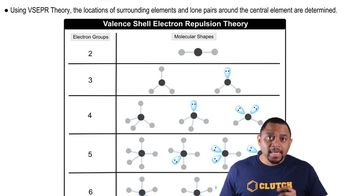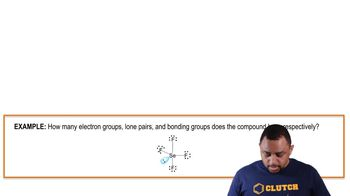Start typing, then use the up and down arrows to select an option from the list.# VSEPR

Pearson
125views
1
INSTRUCTOR: Valence Shell Electron Pair Repulsion, or VSEPR, is a simple yet useful model for predicting molecular shapes. VSEPR has generally three steps or rules to follow when determining the molecular shape. The first step is draw the Lewis structure. The second step is count the number of electron domains. And then the third is determine the shape of the molecule by subtracting out the number of unpaired electrons that do not contribute to the molecular shape. So let's go do a few examples here. Over here, we have beryllium chloride. What do you predict would be the molecular shape for beryllium chloride? So let's go to the board here, and let's go first draw the Lewis structure. So in this case, we have beryllium and it has two electrons, and then it has a chlorine and it has seven electrons, and another chlorine on this side with seven electrons as well. These two electrons are going to form a bond, so the Lewis structure would look something like this. Two chlorines bonded to the beryllium, two electron domains as bonds here, and so these two bonds are going to be as far apart as possible. So this is what the molecular model would look like. It has these two bonds. These are going to be as far away as possible, 180 degrees, so this would be a linear molecule. So let's go do something a little bit more complicated, and let's do the nitrate ion here. So nitrate has an extra electron, so it's an anion here. What would you predict to be the shape of the nitrate ion? So let's go to the board and let's go do the Lewis structure for the nitrate ion. First, we'll start with the nitrogen. Nitrogen has 1, 2, 3, 4, and 5 electrons normally. But this is an ion, an it has an extra one, so we're going to put the extra electron here. And now we have oxygens. Oxygens have 1, 2, 3, 4, and 5, 6 on each one. And they are going to share these two electrons on each of the nitrogens to form a bond. So if we were to draw this Lewis structure, it would look something like this. And we're going to put the ion, the negative charge, there to remember that it's an ion. So we have three electron domains. These are all bonding domains, our bonds. So if we come and draw and determine the model here, we'll notice that they have three domains and these domains are all going to be away as far as possible from each other. And so this is going to be triganol planar, or the bond angle will be 120 degrees between each bond. So let's move on to something a little bit more complicated now, and let's talk about the tetrahedral structure. So we're going to go look at and draw the Lewis structures and determine the shapes for these three molecules, methane, ammonia, and water. So we'll do this a little bit more quickly. And notice that in this case, carbon is going to be bonded to the four hydrogens in this case. Ammonia, this is going to have a lone pair and then be bonded to three hydrogens. And in the case of water, the oxygen is going to have two lone pairs and be bonded to two hydrogens in this case. Notice that the electron domain structure for these three molecules are all the same. They all have four electron domains, four here, four here, three bonds and a lone pair, two bonds and two lone pairs. But these shapes are all going to be very different. Notice here that these are going to form four bonds. These are going to form three bonds. We mentally subtract out this lone pair, and then we mentally subtract out these two lone pairs. And so if we look at these molecules, here's the methane molecule. Notice that it has the four. This is going to be a tetrahedral structure. Four bonds. Each of those bond angles here is going to be 109.5 degrees. In this case, this is the ammonia molecule. Notice we've mentally subtracted out that lone pair, and this is going to be a trigonal pyramidal structure. And the bonding is going to be close to this methane, but this lone pair pushes those bonds a little bit more. And so they're going to be a little bit smaller in size. And finally, we have water which has the two bonds and two lone pairs which are going to squeeze these bonds even a little bit more. So what would you predict to be the order of the bond angles for these three molecules, methane, ammonia, and water, from smallest to largest? So the answer would be that the smallest bond angle is with water. With water, they have the two lone pairs, they're squeezing these bonds the most, and it's going to be the smallest bond angle. Followed by ammonia. In this case, it has one lone pair squeezing this angle a little bit less than 109.5 degrees. And finally, we have the symmetrical methane molecule with the four bonds all with an angle of 109.5 degrees.05:5600:4700:5102:1300:51